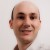# Cp and Cpk values

Six Sigma – iSixSigma Forums Old Forums General Cp and Cpk values

Viewing 3 posts - 1 through 3 (of 3 total)
• Author
Posts
• #31952

Hi,
Can you please anybody tell me how to calculate Cp and Cpk values when mean and standrad deviation is zero for a particular parameter. I do have enough points aroudn 300.
Thanks for any kind of help
A.Sridhar

0
#84809

Hi Sridhar,
If you have around 300 measurements and the standard deviation of these 300 is 0, then you probably have a severe lack of gage discrimination (the ability of the gage to distinguish between parts).  A standard deviation of 0 would mean that all 300 measurements are the same value.  Therefore, you need to acquire a better gage, one that can read to at least one more decimal point than your current one.  By the way, what did your control chart for this process look like?
As you have discovered, a Cp or Cpk index cannot be computed when the standard deviation is 0 because the formulas for these two indices involve dividing by the standard deviation.
Cp = (USL – LSL) / (6 standard deviations)
Since division by 0 is not allowed, you cannot caculate either Cp or Cpk.  As the true process standard deviation approaches 0, then both Cp and Cpk approach plus infinity (which is certainly much better performance than required by 6 sigma!).
Hope this helps.

0
#84810Gabriel
Participant

Mean = 0: No problem. You can calculate Cp and Cpk as you allways do.
Standard deviation = 0: Impossible. This would mean that youe process has no variation, and there is no such a process. From a mathematical point of view, if the variation trends to 0 then Cp trends to infinite and  Cpk tends to infinite if the avrage is within specification and to minus infinite if it is beyond.
Would you explain how did you get a standard deviation = 0? What are you measuring? With which mesurement system?
The first thing that cross my mind is that the measurement system may have a poor resolution to distinguish the differences between parts. For example, if I pack sugar in bags of 1±0.05Kg and measure the quantity with a digital scale with a resolution of 0.01Kg, the fact that I get the same measurement of 1.00Kg from 300 bags does not mean that they actually weight the same. Just that I can not detect the difference which can be of a few grams (0.001Kg).

0
Viewing 3 posts - 1 through 3 (of 3 total)

The forum ‘General’ is closed to new topics and replies.